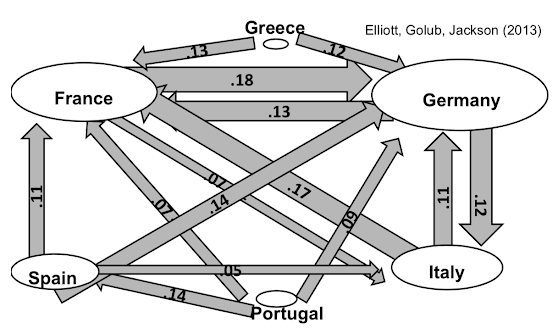# Explore Contagions¶

Simple model of Elliott, Golub Jackson 13:

Companies are linked to each other via various contracts: debts, promised deliries, equity,...

That exposes each company to others' investments and values

First, let's see how to use networks to model exposures

• We are going to work with just sort of straight equity values. But we're just going to first start to see, how it can be that, if I know what percentage of the value of some other company that I am exposed to and I have got a whole of series of these things. How can we keep track of the ultimate exposure that we have in the economy

An organization has direct investments:

• Fraction $c_i$ of value accrues directly to them
• Fraction $1-c_i$ is owed to others

Also hold obligations of $d_i$ other organizations:

• Have claims to those other organizations' investments

# Model¶

• ${1,...,n}$: Organizations (countries, firms, banks...)

• $p_i$: price of investments of organization i

# Cross Holdings¶

• $C_{ij}$: cross holdings: fraction of org j owned by org i

• $C_{ii} = 0$: (don't own yourself)

• $\hat C_{ii} = 1 - \sum_j C_{ji}$: fraction of org i privately held

# Value of an Organization¶

book value:

$$V_i = p_i + \sum_j C_{ij}V_j$$

• $p_i$: direct asset holdings
• $\sum_j C_{ij}V_j$: cross-holdings

Vectorize: $$V = P + CV$$

Leontief calculation of book value $$V = (I - C)^{-1}P$$

Market value-value to final (private) investors.

$v_i = \hat C_{ii}V_i$

$v = \hat C(I - C)^{-1}p$

$v = Ap$

• $A_{ij}$: fraction of the investments owned by org j that ultimately accrue to private shareholders of i

# Example¶

• Two organizations: n = 2

• Each owns half of the other: $C = \begin{vmatrix} 0 & 0.5 \\ 0.5 & 0 \end{vmatrix}$

• Implied holdings by private investors: $\hat C = \begin{vmatrix} 0.5 & 0 \\ 0 & 0.5 \end{vmatrix}$

• Final investors' claims on assets: $A = \hat C(I-C)^{-1} = \begin{vmatrix} 2/3 & 1/3 \\ 1/3 & 2/3 \end{vmatrix}$

A means that 2/3 of the value of the investments of organization "1", actually end up going to the owners of organiazation "1", and 1/3 of organization "2" goes to organization "1"'s owners, and vice versa

Basic structure in terms of the ownership:Let's figure out where this 2/3, 1/3 came from:

What happens to \\$1 of investment income to 1?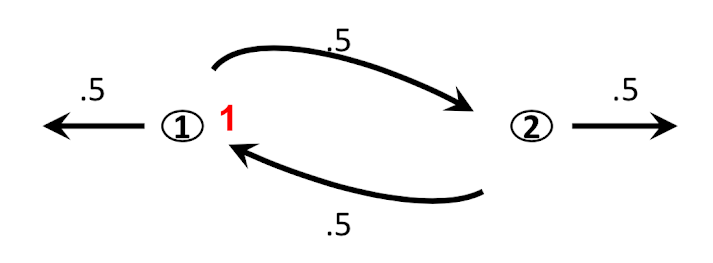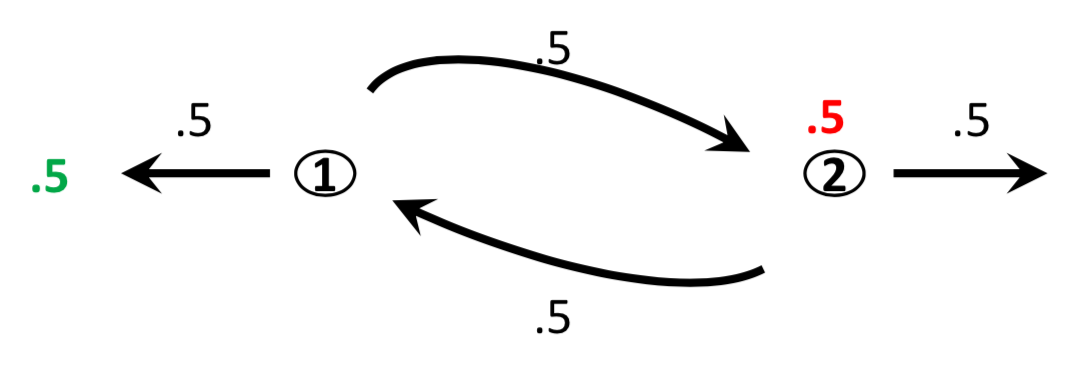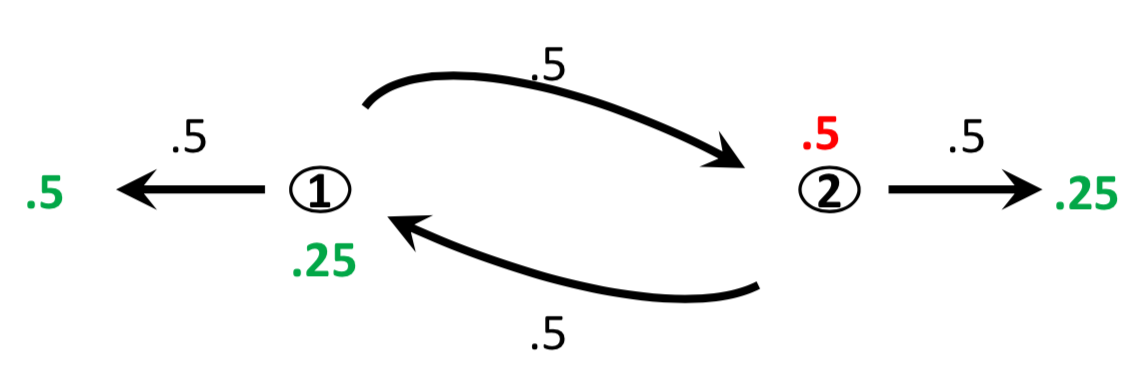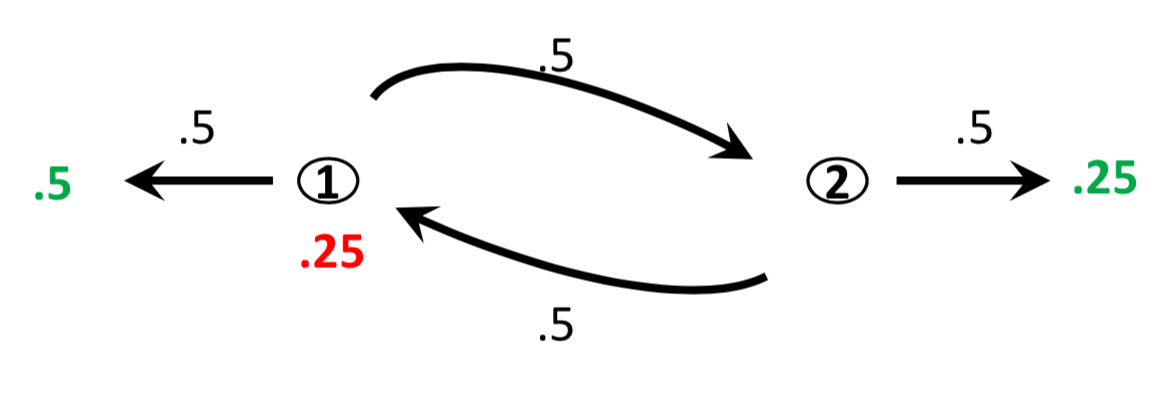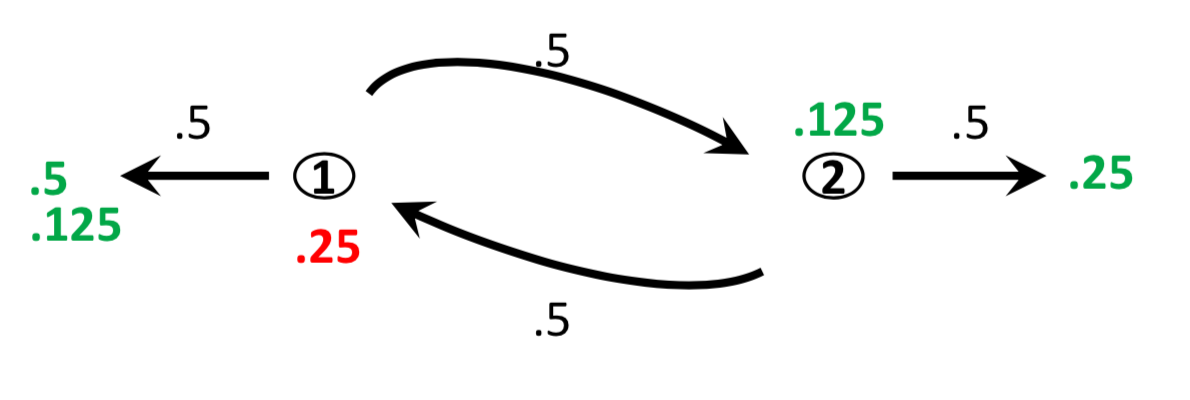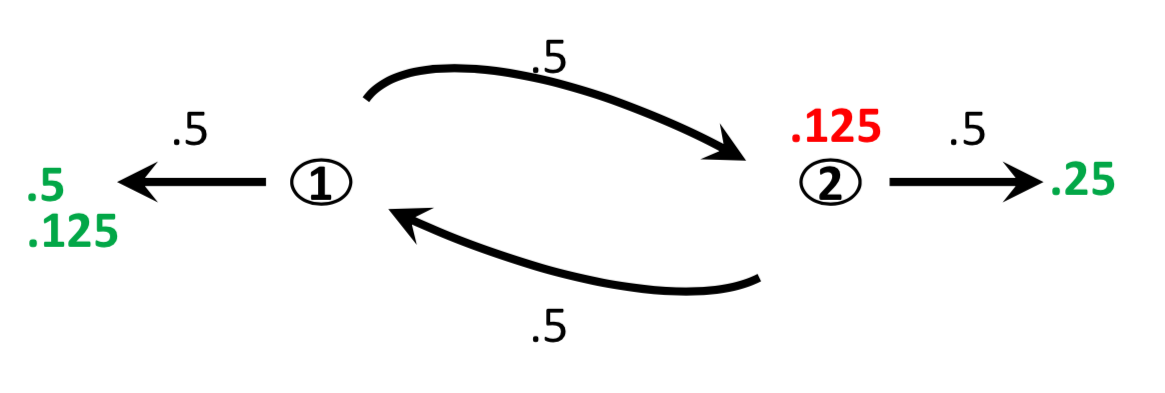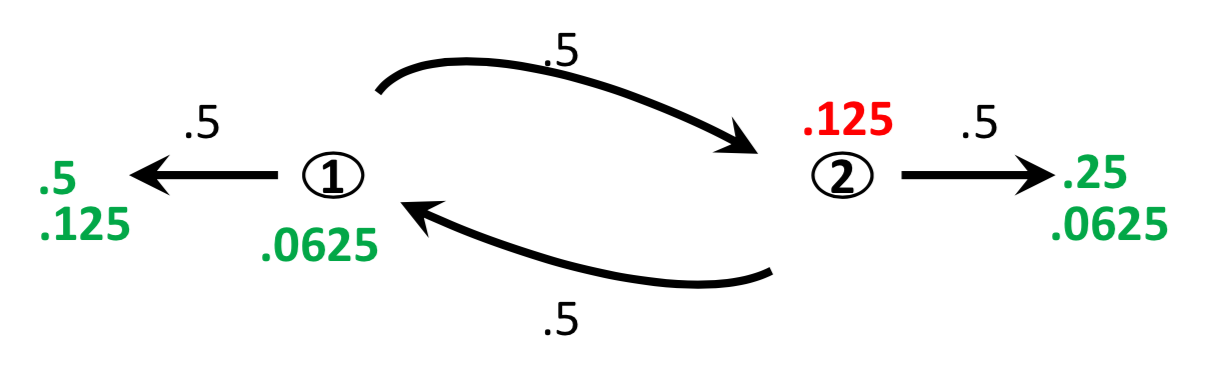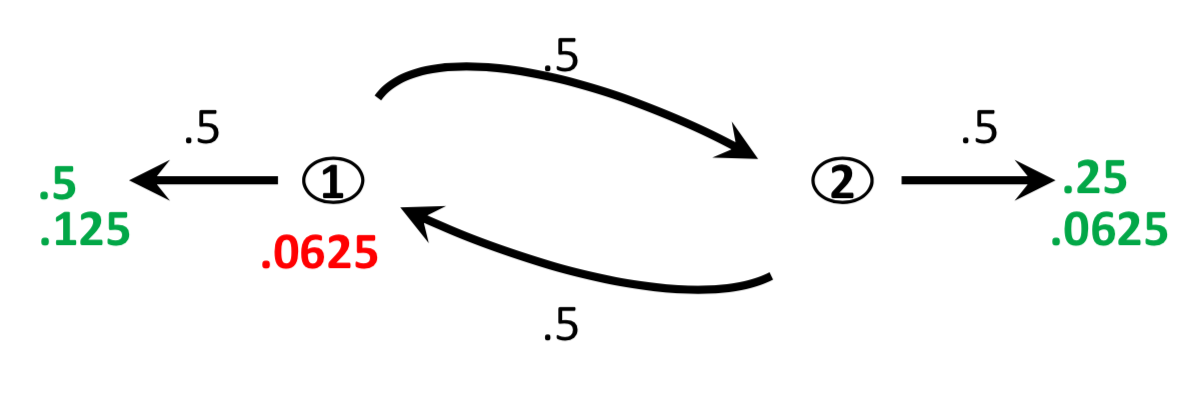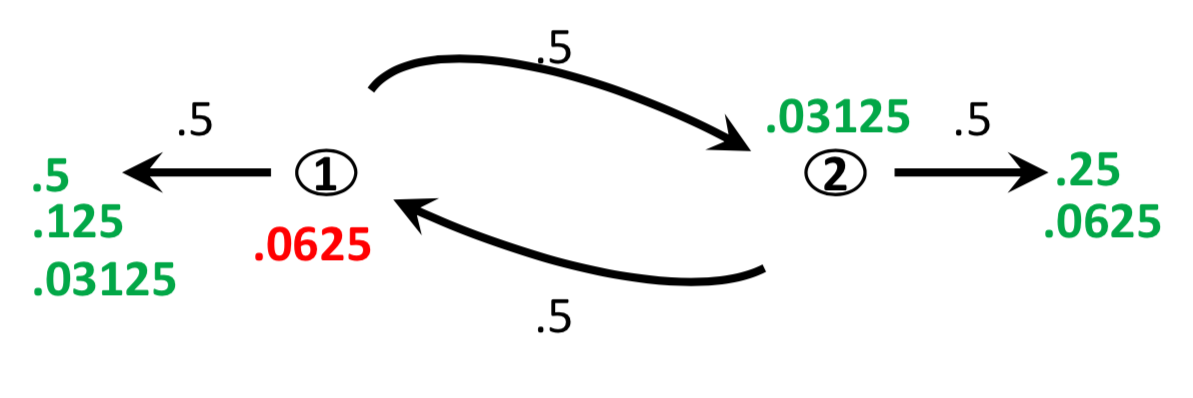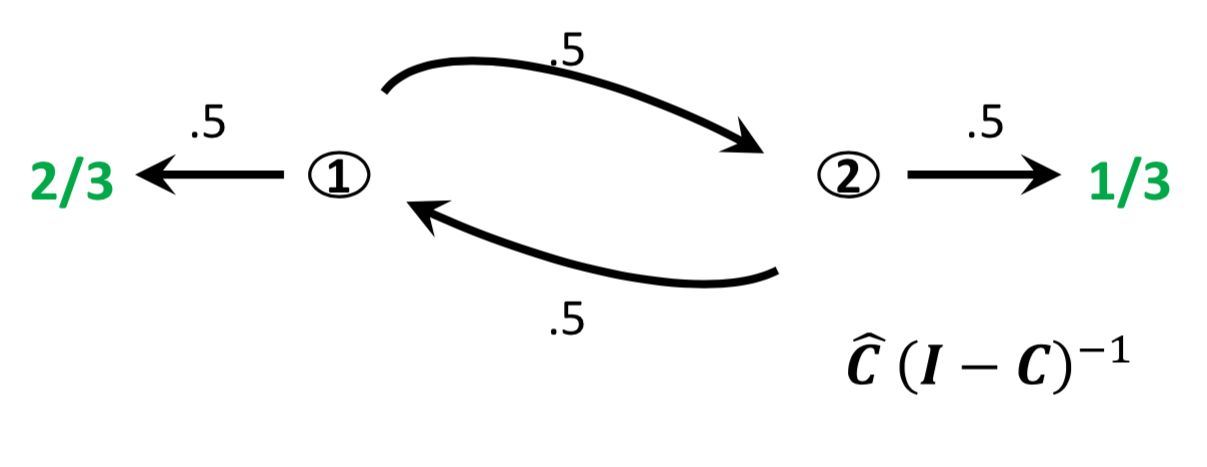as you keep iterating this, eventually 2/3 of that went out one side, and 1/3 came out the other side, and that was exactly the calculation we got for the "A" matrix.